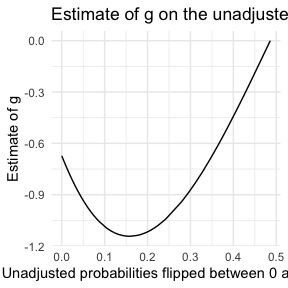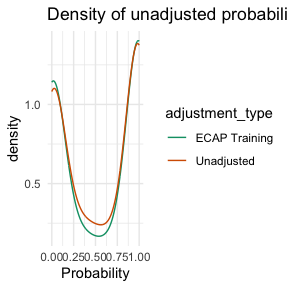# Implementing the Excess Certainty Adjusted Probability (ECAP) procedure

This is an R package for implementing the ECAP method as described in the paper “Irrational Exuberance: Correcting Bias in Probability Estimates” by Gareth M. James, Peter Radchenko, and Bradley Rava. For details, please consult the paper at http://faculty.marshall.usc.edu/gareth-james/Research/Probs.pdf.

# Introduction

It is becoming ever more common to observe probability estimates for a large number of events, such as default risks for numerous bonds. Unfortunately, even with unbiased estimates, selecting events corresponding to the most extreme probabilities can result in systematically underestimating the true level of uncertainty. This package implements an empirical Bayes approach “Excess Certainty Adjusted Probabilities” (ECAP), using a variant of Tweedie’s formula, which updates probability estimates to correct for selection bias. ECAP is a flexible non-parametric method, which directly estimates the score function associated with the probability estimates, so it does not need to make any restrictive assumptions about the prior on the true probabilities. ECAP also works well in settings where the probability estimates are biased.

## What does the package do?

This R package implements the ECAP adjustment procedure on a vector of probability estimates.

## How is the package structured?

The package has one main function, “ecap”. This takes in a vector of unadjusted probability estimates that you already have the results for. That information is used to create the ecap model that can be passed into the “predict” function. “predict” only needs the ecap object and a vector of unadjusted probability estimates that you want ECAP corrected. The result is a vector of ECAP adjusted probability estimates that correspond to the given unadjusted ones. The adjustment allows the user to decide if the bias should be estimated or not.

# Installing the package

The ECAP package is available on github and can be installed through the “devtools” package.

library(devtools)
install_github("bradleyrava/ecap")

Once installed, you can load the package and functions into your R session with the following command

library(ecap)

# Example

For guidance and reproducibility, this package includes the FiveThirtyEight data analyzed in the paper. It was originally accessed from FiveThirtyEight’s github https://github.com/fivethirtyeight/data/tree/master/forecast-review.

Let’s load the classic estimates from the FiveThirtyEight 2018 Midterm Election Predictions into our environment.

x <- ecap::elections_2018
p_obs <- x$Democrat_WinProbability[x$version=="classic"]
win_var <- x$Democrat_Won[x$version=="classic"]

This gives us a vector of unadjusted probabilities and their corresponding wins and losses. In reality, the probabilities represent FiveThirtyEight’s estimate of a given race that occurred in the US 2018 Midterm Elections. The win variable is the eventual outcome of that race.

Let’s take half of these probabilities to train our model and the other half to predict.

set.seed(1)
train_rows <- sample(1:length(p_obs), length(p_obs)/2)
test_rows <- (1:length(p_obs))[-train_rows]

p_obs_train <- p_obs[train_rows]
win_var_train <- win_var[train_rows]

p_obs_test <- p_obs[test_rows]

We can now fit our ECAP model on the training probabilities / wins and losses. The win_id variable tells the function what a “win” looks like in your data. Typically a win is represented as a 1 (meaning the event occurred) and a loss as a 0 (the event did not occur).

As discussed in the paper, we suspect that the FiveThirtyEight probabilities have bias in them. So we set bias_indicator=T in order to allow the method to estimate the bias.

ecap_fit <- ecap(unadjusted_prob = p_obs_train, win_var = win_var_train, win_id = 1, bias_indicator = T)

To inspect the object, we can get a quick look inside by calling print. If you want a deeper summary, call the summary function on the ecap object.

print(ecap_fit)
##
##  E(p|p_tilde) + Var(p|p_tilde) / E(p|p_tilde)
##
##  The optimal parameters picked for the ECAP adjustment are: lambda = 3e-04,  gamma = 0.001 and theta =  2
summary(ecap_fit)
##
##
##  The optimal parameters picked for the ECAP adjustment are: lambda = 3e-04,  gamma = 0.001 and theta =  2
##
##  The optimal parameters were picked from these grids (*** estimate hit end of grid)
##  Lambda Grid - Length 13 From 0 to 1
##  Gamma Grid - Length 50 From 0.001 to 0.05 ***
##  Theta Grid - Length 61 From -4 to 2 ***
##
##  The unadjusted probabilities range from 0 to 1 with an average of 0.5452  - Length 253
##  The ECAP training probabilities range from 0 to 1 with an average of 0.5427  - Length 253

We also include diagnostics when you plot an ecap object. The details of which are described in the paper, but if you are interested in seeing what the estimate of the function $$g(\tilde{p})=\tilde{p}(1-\tilde{p})\log f(\tilde{p})$$ (where $$\tilde{p}$$ represents our unadjusted probabilities) looks like, you can call plot on an ecap object. This is returned as the first plot and the second one compares the training unadjusted probability estimates to the adjusted ecap ones.

plot(ecap_fit)
## []##
## []Finally, we can use this ecap object to adjust a new set of probabilities. In reality, these probabilities represent events that have not occurred yet, so the only thing the predict function needs is the ecap model and a vector of new unadjusted probability estimates.

prob_ecap <- predict(object=ecap_fit, new_unadjusted=p_obs_test)
head(cbind.data.frame(ecap_p=prob_ecap, unadjusted_p=p_obs_test))
##         ecap_p unadjusted_p
## 1 1.110181e-03      0.01692
## 2 8.926602e-06      0.00062
## 3 6.851260e-04      0.01280
## 4 9.993809e-01      0.98794
## 5 9.919495e-01      0.94970
## 6 8.845578e-01      0.78996

# Further Functionality

If you have any further questions about the functions, please look at the individual documentation for each function. “?ecap”. Specifically, if needed you can adjust the grid of tuning parameters that the package uses to pick the parameters needed to do the ECAP adjustment. A good sign you should do this is if the function is picking a value that is at the end of your grid. To know if this is happening, look at the summary output of your ecap object.

## Included Data

For reproducibility, this package includes the FiveThirtyEight dataset discussed in the paper along with ESPN’s win probabilities. In particular, the ESPN dataset has the win probability estimates that occured closest to the given timestamps at the end of every games 4th quarter in the 2016 and 2017 NCAA football seasons.

To access this data, simply run the following commands. Where $$x$$ is the FiveThirtyEight 2018 midterm election data and $$y$$ is the ESPN NCAA football win probabilities.

x <- ecap::elections_2018
y <- ecap::espn